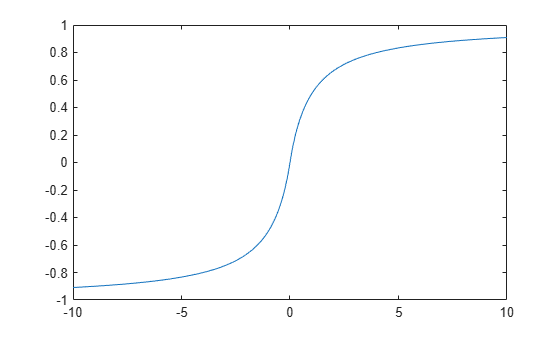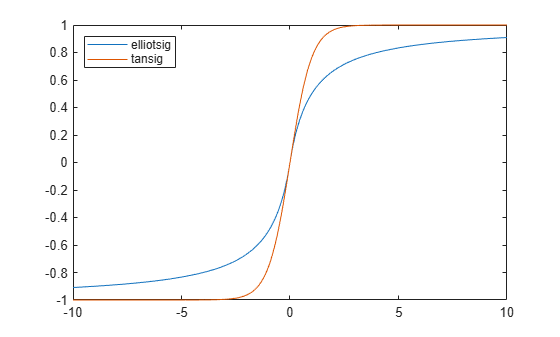## Optimize Neural Network Training Speed and Memory

### Memory Reduction

Depending on the particular neural network, simulation and gradient calculations can occur in MATLAB® or MEX. MEX is more memory efficient, but MATLAB can be made more memory efficient in exchange for time.

To determine whether MATLAB or MEX is being used, use the `'showResources'` option, as shown in this general form of the syntax:

`net2 = train(net1,x,t,'showResources','yes')`

If MATLAB is being used and memory limitations are a problem, the amount of temporary storage needed can be reduced by a factor of `N`, in exchange for performing the computations `N` times sequentially on each of `N` subsets of the data.

`net2 = train(net1,x,t,'reduction',N);`

This is called memory reduction.

### Fast Elliot Sigmoid

Some simple computing hardware might not support the exponential function directly, and software implementations can be slow. The Elliot sigmoid `elliotsig` function performs the same role as the symmetric sigmoid `tansig` function, but avoids the exponential function.

Here is a plot of the Elliot sigmoid:

```n = -10:0.01:10; a = elliotsig(n); plot(n,a)```Next, `elliotsig` is compared with `tansig`.

```a2 = tansig(n); h = plot(n,a,n,a2); legend(h,'elliotsig','tansig','Location','NorthWest')```To train a neural network using `elliotsig` instead of `tansig`, transform the network’s transfer functions:

```[x,t] = bodyfat_dataset; net = feedforwardnet; view(net) net.layers{1}.transferFcn = 'elliotsig'; view(net) net = train(net,x,t); y = net(x)```

Here, the times to execute `elliotsig` and `tansig` are compared. `elliotsig` is approximately four times faster on the test system.

```n = rand(5000,5000); tic,for i=1:100,a=tansig(n); end, tansigTime = toc; tic,for i=1:100,a=elliotsig(n); end, elliotTime = toc; speedup = tansigTime / elliotTime speedup = 4.1406```

However, while simulation is faster with `elliotsig`, training is not guaranteed to be faster, due to the different shapes of the two transfer functions. Here, 10 networks are each trained for `tansig` and `elliotsig`, but training times vary significantly even on the same problem with the same network.

```[x,t] = bodyfat_dataset; tansigNet = feedforwardnet; tansigNet.trainParam.showWindow = false; elliotNet = tansigNet; elliotNet.layers{1}.transferFcn = 'elliotsig'; for i=1:10, tic, net = train(tansigNet,x,t); tansigTime = toc, end for i=1:10, tic, net = train(elliotNet,x,t), elliotTime = toc, end ```